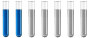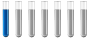## I need help with finding molar ratio

Chemistry and homework help forum.

Organic Chemistry, Analytical Chemistry, Biochemistry, Physical Chemistry, Computational Chemistry, Theoretical Chemistry, High School Chemistry, Colledge Chemistry and University Chemistry Forum.

Share your chemistry ideas, discuss chemical problems, ask for help with scientific chemistry questions, inspire others by your chemistry vision!

Please feel free to start a scientific chemistry discussion here!

Discuss chemistry homework problems with experts!

Ask for help with chemical questions and help others with your chemistry knowledge!

Moderators: Xen, expert, ChenBeier

ndgkhang
Jr. MemberPosts: 5
Joined: Mon Jun 20, 2022 10:36 am

### I need help with finding molar ratio

I am currently using Ca(NO3)2*4H2O and P2O5 and I need to have the molar ratio of Ca/P = 1.5
I wonder how could I do that?

ChenBeier
Distinguished MemberPosts: 1420
Joined: Wed Sep 27, 2017 7:25 am
Location: Berlin, Germany

### Re: I need help with finding molar ratio

You need 1 mol of the Calcium nitrate tetra hydrate and 0.333 mol of the phosphorous Penta oxide.

One 1 mol Ca(NO3)2*4H2O correspond to 1 mol Ca
and 1 mol P2O5 correspond to 2 mol P

Ratio Ca/P = 1.5 = 1/0,666 mol = 1 Ca(NO3)2*4H2O /0,333 P2O5

With the molar mass of each component you can calculate the mass in g.
ndgkhang
Jr. MemberPosts: 5
Joined: Mon Jun 20, 2022 10:36 am

### Re: I need help with finding molar ratio

ChenBeier wrote: Mon Jun 20, 2022 11:35 am You need 1 mol of the Calcium nitrate tetra hydrate and 0.333 mol of the phosphorous Penta oxide.

One 1 mol Ca(NO3)2*4H2O correspond to 1 mol Ca
and 1 mol P2O5 correspond to 2 mol P

Ratio Ca/P = 1.5 = 1/0,666 mol = 1 Ca(NO3)2*4H2O /0,333 P2O5

With the molar mass of each component you can calculate the mass in g.
Hi there,

Thank you very much for your reply, I found out I need to mix 236.15g of Calcium Nitrate Tera Hydrate with 94.53537g of Phosphorous Penta Oxide ratio in order to produce Ca/P = 1.5

Another quick question, there's this literature I have been reading and it suggested me to dissolve Ca(NO3)2*4H2O and P2O5 in absolute ethanol to form 0.01 mol and 0.003 mol solution. I dont understand that sentence, could you help explain what does it mean to dissolve down to 0.01 mol and 0.003 mol, and does that have anything to do with the mass I just found by your help?

Thank you again for your help.
ChenBeier
Distinguished MemberPosts: 1420
Joined: Wed Sep 27, 2017 7:25 am
Location: Berlin, Germany

### Re: I need help with finding molar ratio

The calculation for P2O5 is wrong its only 47,328 g

Molar weight of P2O5 is 142 g/ mol. Multiplied with 0.3333 is 47.3286 g

1 mol to 0.333 mol can also diluted down

In this case 0.01 mol to 0.0033 mol

What means in mass 2,36 g Calcium Nitrate tetra hydrate and 0,473 g Phosphorous penta oxide
ndgkhang
Jr. MemberPosts: 5
Joined: Mon Jun 20, 2022 10:36 am

### Re: I need help with finding molar ratio

ChenBeier wrote: Mon Jun 20, 2022 2:03 pm The calculation for P2O5 is wrong its only 47,328 g

Molar weight of P2O5 is 142 g/ mol. Multiplied with 0.3333 is 47.3286 g

1 mol to 0.333 mol can also diluted down

In this case 0.01 mol to 0.0033 mol

What means in mass 2,36 g Calcium Nitrate tetra hydrate and 0,473 g Phosphorous penta oxide
Thank you for the correction, I was looking at the P4O10 Molar mass.
For the dissolve in absolute ethanol to form 0.01 mol (Calcium Nitrate Tetrahydrate) and 0.003 mol (phosphorous pentoxide), which equation I can use to find how much mass in how much volume of ethanol to dissolve to desire mol?
ChenBeier
Distinguished MemberPosts: 1420
Joined: Wed Sep 27, 2017 7:25 am
Location: Berlin, Germany

### Re: I need help with finding molar ratio

It is the same equation like above. I wrote the result already. It is only 1/100 of the calculated value.

Moles = mass/ Molar mass, n = m/M

In case of P4O10 is the result more worse, because 1 mol P4O10 = 4 mol P

It is more worse then 1/0.166666 Ca/P4O10 = 1/4*0,16666 Ca/P = 1/0,66666 = 1.5

The mass will be still the same either calculated as P2O5 or P4O10 47,3 g.

The volume is calculated to 1 Liter. Concentration normaly given as mol/l.
ndgkhang
Jr. MemberPosts: 5
Joined: Mon Jun 20, 2022 10:36 am

### Re: I need help with finding molar ratio

ChenBeier wrote: Mon Jun 20, 2022 10:12 pm It is the same equation like above. I wrote the result already. It is only 1/100 of the calculated value.

Moles = mass/ Molar mass, n = m/M

In case of P4O10 is the result more worse, because 1 mol P4O10 = 4 mol P

It is more worse then 1/0.166666 Ca/P4O10 = 1/4*0,16666 Ca/P = 1/0,66666 = 1.5

The mass will be still the same either calculated as P2O5 or P4O10 47,3 g.

The volume is calculated to 1 Liter. Concentration normaly given as mol/l.
Hi there,

As long as I use Ca/P = 1.5 it would work out well, right? either: 1/0.333 or, 0.01/0.0033, etc... But since the literature suggested to dissolved down to 0.01 and 0.0033 in absolute ethanol, Ca and P respectively. It means that I would need to dissolve 2.36g Ca(NO3)2*4H2O in 1L of absolute ethanol and 0.473g of P2O5 in 1L of absolute ethanol. Do you have any idea why the literature suggested me to dissolve down to 0.01 and 0.0033 mol instead of 1 and 0.33 mol in ethanol? Would it be more concentrated but since the Ca/P is the same ratio, it would work out as well?

Quoted Synthesis of B-tricalcium phosphate by H. Chaair, H. Labjar , O. Britel
"Calcium nitrate tetrahydrate (Ca(NO)2 4H2O, Merck) and phosphorus pentoxide (P, Merck) were selected as Ca and P precursors, respectively. A design amount of Ca(NO3), 4 H2O and P2O5 was dissolved in absolute ethanol to form 0.01 mol and 0.003 mol solution, respectively. The solutions were mixed as initial mixed precursor solution and were continuously stirred for 30 minutes at ambient temperature until a white transparent gel was obtained."
ChenBeier
Distinguished MemberPosts: 1420
Joined: Wed Sep 27, 2017 7:25 am
Location: Berlin, Germany

### Re: I need help with finding molar ratio

Its only a diluted solution nothing else. You can choose any amount only to achieve Ca/P = 1.5. Maybe its also a matter of soloubility. I would go according the receipe.
ndgkhang
Jr. MemberPosts: 5
Joined: Mon Jun 20, 2022 10:36 am

### Re: I need help with finding molar ratio

ChenBeier wrote: Tue Jun 21, 2022 10:58 am Its only a diluted solution nothing else. You can choose any amount only to achieve Ca/P = 1.5. Maybe its also a matter of soloubility. I would go according the receipe.
Thank you for your help. I will try it out according to the recipe
emmausa
NewbiePosts: 3
Joined: Tue Oct 17, 2023 7:36 pm

### Re: I need help with finding molar ratio

To achieve a molar ratio of Ca/P = 1.5, you would need to use approximately 0.9 grams of Ca(NO3)2·4H2O for every 1 gram of P2O5. This ratio would give slither io you the desired molar ratio of 1.5.